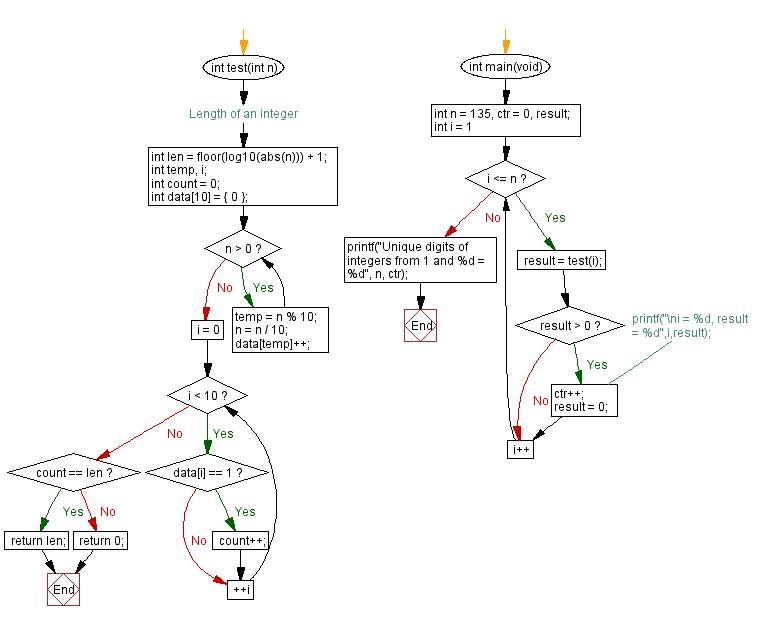﻿ C - Count unique digits of integers

# C Programming: Count unique digits of integers

## C Programming Mathematics: Exercise-37 with Solution

Write a C program that counts the number of integers whose digits are unique from 1 and a given integer value.

Example:
Input: n = 30
From 1 to 30 all the integers have unique digits, except 11 and 22
Output: 28

Test Data:
(30) -> 28
(135) -> 110

Sample Solution:

C Code:

``````#include <stdio.h>
#include <math.h>

int test(int n) {
//Length of an integer
int len = floor(log10(abs(n))) + 1;
int temp, i;
int count = 0;
int data = {
0
};
while (n > 0) {
temp = n % 10;
n = n / 10;
data[temp]++;
}
for (i = 0; i < 10; ++i) {
if (data[i] == 1)
count++;
}
if (count == len)
return len;
else
return 0;
}
int main(void) {
int n = 135, ctr = 0, result;
for (int i = 1; i <= n; i++) {
result = test(i);
if (result > 0) {
//printf("\ni = %d, result = %d",i,result);
ctr++;
result = 0;
}
}
printf("Unique digits of integers from 1 and %d = %d", n, ctr);
}

```
```

Sample Output:

```Unique digits of integers from 1 and 135 = 110
```

Flowchart:C Programming Code Editor:

Improve this sample solution and post your code through Disqus.

What is the difficulty level of this exercise?

Test your Programming skills with w3resource's quiz.

﻿